19 January 2018

# Creating a photonic crystal using a GDS array

### Make use of GDS array instantiation

```# example created by Bright Photonics

import nazca as nd

with nd.Cell('hole') as hole:
nd.Polygon(points=hole_shape).put(0)

hole.put(array=[20, [0.25, 0], 30, [0, 0.30]])
hole.put(5, -5, array=[40, [0.20, 0.10], 50, [0.10, 0.30]])

nd.export_gds()```

In this example we show how to create a photonic crystal. We use Nazca geometries library to define a hole shape, which we then put in the layout using GDS array.

The first array is composed of 20 x 30 hole-shapes spaced by 250 nm in x-direction and 300 nm in y-direction. In the second array of 40 x 50 hole-shape we introduce additional translation of 100 nm x 100 nm. The format of the array is as: [col#, [dx1, dy1], row#, [dx2, dy2]]. Note: to rotate the array you have to play with the two displacement vectors [dx1, dy1] and [dx2, dy2]. If you rotate the original shape, then the original shape is rotated within the lattice.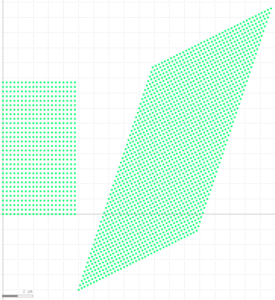We can vary the diameter of the holes and the holes finesse by, for example, creating a function with the two variables.

```# example created by Bright Photonics

import nazca as nd

def hole(diameter=0.1, points=8):
with nd.Cell('hole') as cell:
nd.Polygon(points=hole_shape).put(0)
return cell

hole().put(array=[20, [0.25, 0], 30, [0, 0.30]])
hole(diameter=0.2, points=36).put(5, -5, array=[40, [0.20, 0.10], 50, [0.10, 0.30]])

nd.export_gds()```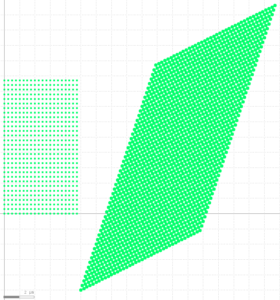Finally, we can specify a layer number in which our structures should be placed.

```# example created by Bright Photonics

import nazca as nd

def hole(diameter=0.1, points=8, layer=None):
with nd.Cell('hole') as cell:
nd.Polygon(points=hole_shape, layer=layer).put(0)
return cell

hole().put(array=[20, [0.25, 0], 30, [0, 0.30]])

hole(diameter=0.2, points=36, layer=1).\
put(5, -5, array=[40, [0.20, 0.10], 50, [0.10, 0.30]])

hole(diameter=0.1, points=4, layer=2).\
put(5, -5, array=[40, [0.20, 0.10], 50, [0.10, 0.30]])

nd.export_gds()```

We used demofab_klayout_colors.lyp to view the masks in KLayout.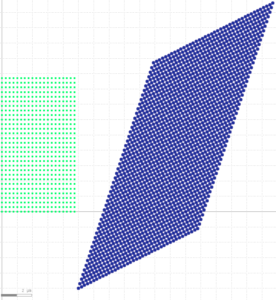## Related Tutorials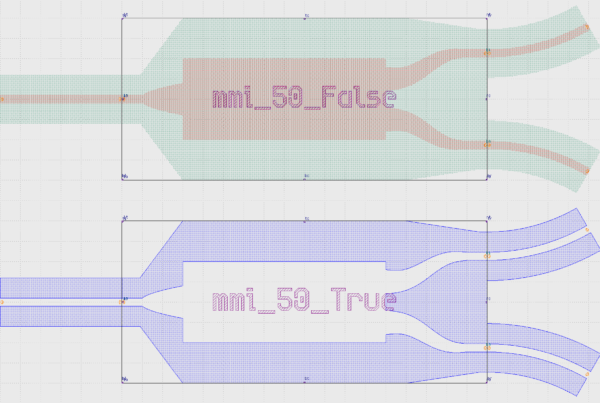In this example we show how to create an inverted MMI with custom interconnects.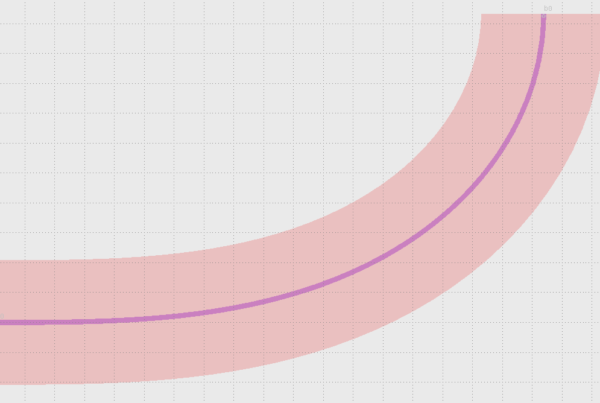In this example we show how to use Euler bends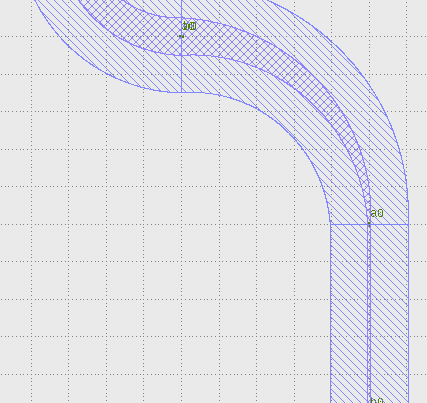In this example we show how to create a free form parametric curve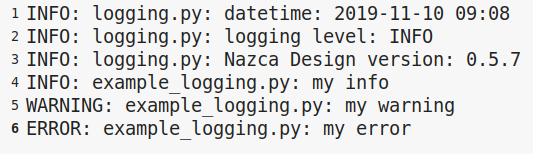In this example we show how to log your layout.